Question

# Question 3 1p What is the integrating factor for xy-3y=x^2? x^(-3) -3/x O e^(-3/) O e^(1/2x^2)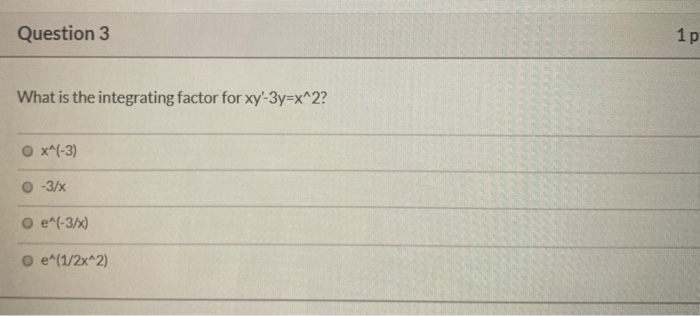Question 3 1p What is the integrating factor for xy-3y=x^2? x^(-3) -3/x O e^(-3/) O e^(1/2x^2)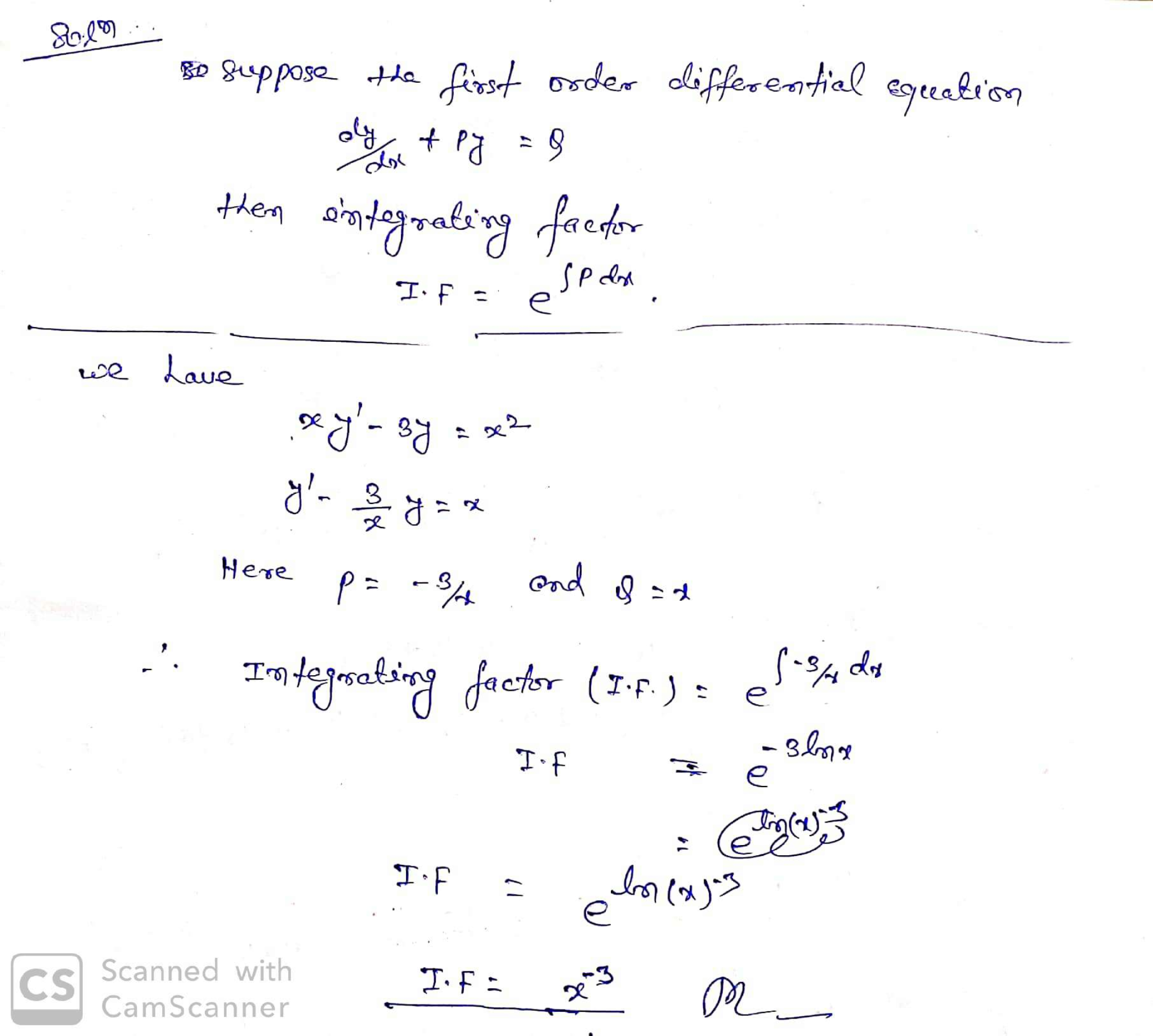#### Earn Coins

Coins can be redeemed for fabulous gifts.

Similar Homework Help Questions
• ### QUESTION 22 Find an integrating factor of the form X"y" and solve the equation. (2x+4y2-9y)dx+ (3y-6x)...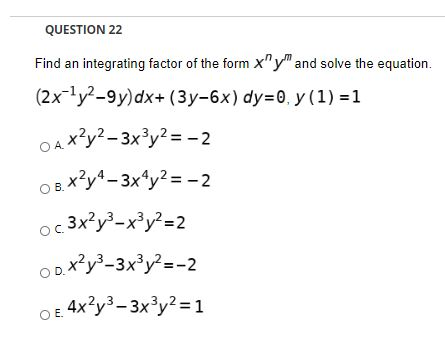QUESTION 22 Find an integrating factor of the form X"y" and solve the equation. (2x+4y2-9y)dx+ (3y-6x) dy=0, y (1) =1 O A *?y2 – 3x3y2 = -2 08.x2y4 – 3x4y2 = -2 oc 3x²y3 – x3y2=2 00.x2y3–3x3y2=-2 o e 4x2y3 – 3x3y2 = 1

• ### QUESTION 25 Find an integrating factor of the form x"y" and solve the equation. (2x+y2-9y)dx+ (3y-6x)...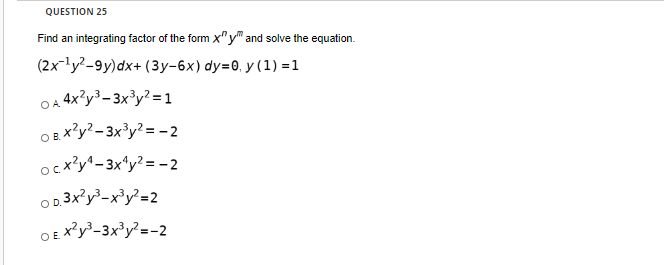QUESTION 25 Find an integrating factor of the form x"y" and solve the equation. (2x+y2-9y)dx+ (3y-6x) dy=0; y(1) =1 A 4x2y3 – 3x3y2 = 1 o e x?y2-3x3y2 = -2 ocx?y* - 3x^y2=-2 02.3x2y3 – x3y2=2 Ex?y-3x?y2=-2

• ### Is there a systematic way to determine an integrating factor #mu(x,y)# of the form #x^n y^m#, given a not-necessarily-exact differential equation?

My book covers special integrating factors #mu# that are functions of only #x# or only #y#, but kinda glosses over how to find an integrating factor that is a function of #x# AND #y#. Example equation: #(2y^2 - 6xy)dx + (3xy - 4x^2)dy = 0# The integrating factor was #mu(x,y) = xy#, and the solution was #F(x,y) = x^2y^3 - 2x^3y^2 = C#. I was able to figure out what the integrating factor was, and solve the equation, but I...

• ### 1. xy' = 3y + 3 3. (x + 1)y' - (2x + 3) y =...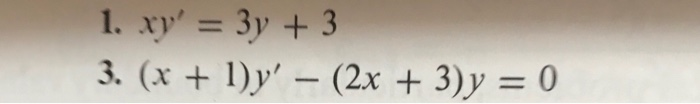1. xy' = 3y + 3 3. (x + 1)y' - (2x + 3) y = 0

• ### Find an integrating factor of the form X"y" and solve the equation. (2x-172-9y)dx + (3y-6x) dy=0,...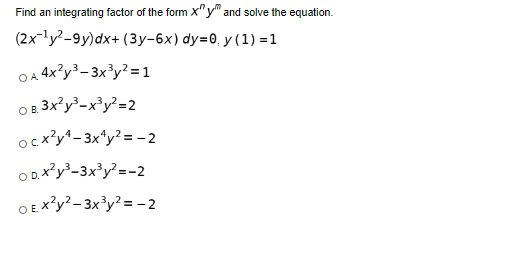Find an integrating factor of the form X"y" and solve the equation. (2x-172-9y)dx + (3y-6x) dy=0, y(1) =1 OA 4x2y3 – 3x3y2 = 1 08.3x2y3 – x3y2=2 ocx?y* - 3x4y2 = -2 D.*?y3 - 3x3y2=-2 Ex?y2 – 3x3y2 = -2

• ### 1P Question 3 1 Evaluate the double integral: SS sin?(x) dx2 7 o (+ cos(2x)) 0}...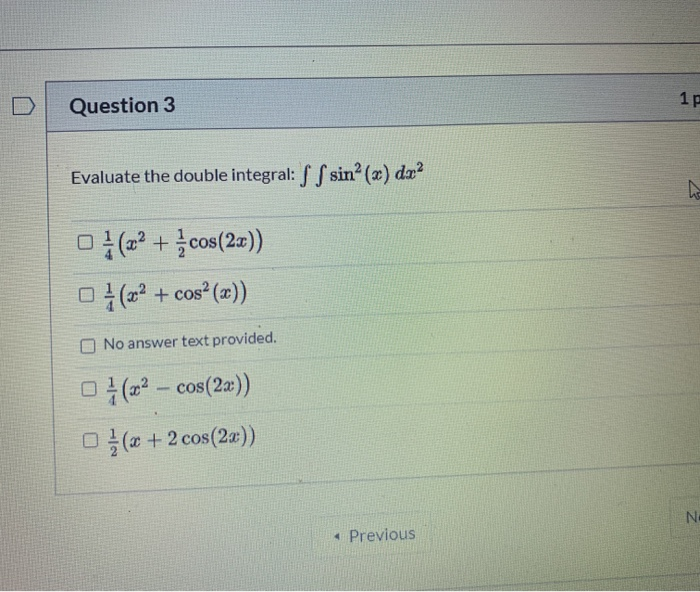1P Question 3 1 Evaluate the double integral: SS sin?(x) dx2 7 o (+ cos(2x)) 0} (x2 + cosº (x)) No answer text provided. 0}(– cos(2x)) 0} (x + 2 cos(2x)) NE Previous

• ### Identify the equation as separable, linear, exact, or having an integrating factor that is a function...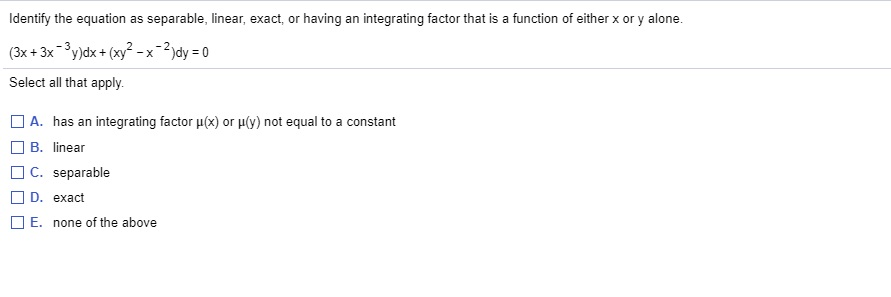Identify the equation as separable, linear, exact, or having an integrating factor that is a function of either x or y alone (3x+3x - 3y)dx + (xy? - x-2)dy = 0 Select all that apply. A. has an integrating factor p(x) or p(y) not equal to a constant OB. linear OC. separable D. exact E. none of the above

• ### 4. specify which letter answer 4) An integrating factor for the lirear differential equation Xy't xy=1...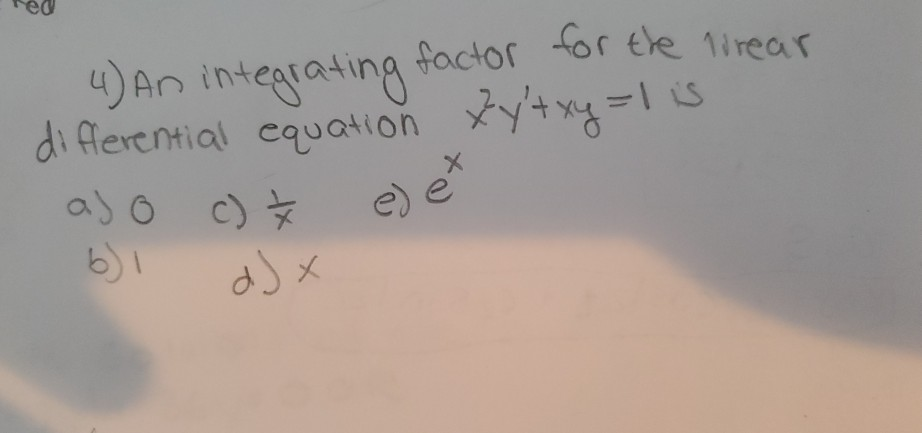4. specify which letter answer 4) An integrating factor for the lirear differential equation Xy't xy=1 is aj o c) e) et dJ x

• ### Find an integrating factor for (2xy^2)dx + (2x^2y+x^2y^2)dy = 0

Find an integrating factor for (2xy^2)dx + (2x^2y+x^2y^2)dy = 0

• ### Identify the equation as separable, linear, exact, or having an integrating factor that is a function...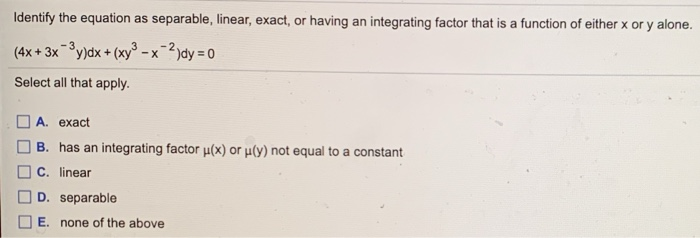Identify the equation as separable, linear, exact, or having an integrating factor that is a function of either x or y alone. (4x+3x - 3y)dx + (xy3 – x-2)dy = 0 Select all that apply. A. exact B. has an integrating factor u(x) or (y) not equal to a constant C. linear D. separable E. none of the above